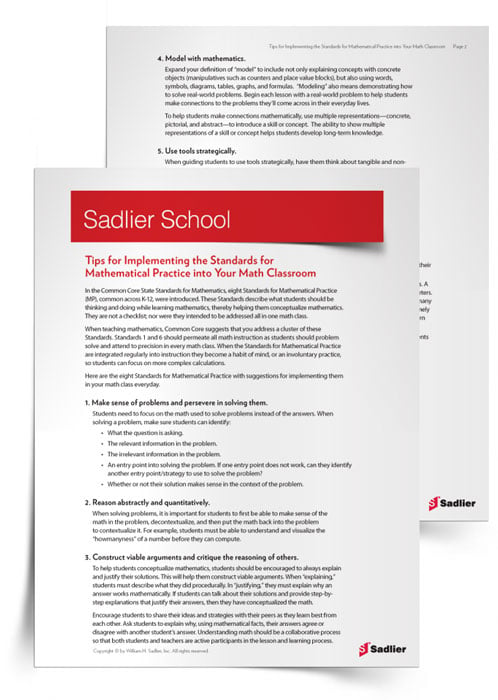1.800.221.5175
Core Program
Full Access
Core Program
Full Access
Core Program
Full Access
National Program
Full Access
New York
Critical Thinking for Active Math Minds
Preparing for Standards Based Assessments
Vocabulary Workshop, Tools for Comprehension Grades 1–5
Print Program
Interactive Edition
Print Program
Interactive Edition
Vocabulary Workshop, Tools for Excellence Grades 6–12+
Print Program
Interactive Edition
Vocabulary for Success
Progress English Language Arts Grades K–8
National Program
Full Access
New York
Grammar Workshop, Tools for Writing
Grammar for Writing
Writing a Research Paper
Writing Workshop
Print Program
Interactive Edition

A K–8 resource to support deep comprehension of math skills and conceptsTopics:

#### July 2, 2020 mp-abstract-quantitative, mp-reasoning, mp-problem-solving, mp-repeated-reasoning, mp-modeling, mp-using-tools, mp-precision, mp-structure

Summer break is the perfect time for teachers to relax, reflect on the past year, complete PD hours, and find amazing math printables for their teaching toolbox. In this article, you'll find 12 printable Mathematical Practices PDF resources that will assist in implementing the Standards in your classroom (or virtual classroom)!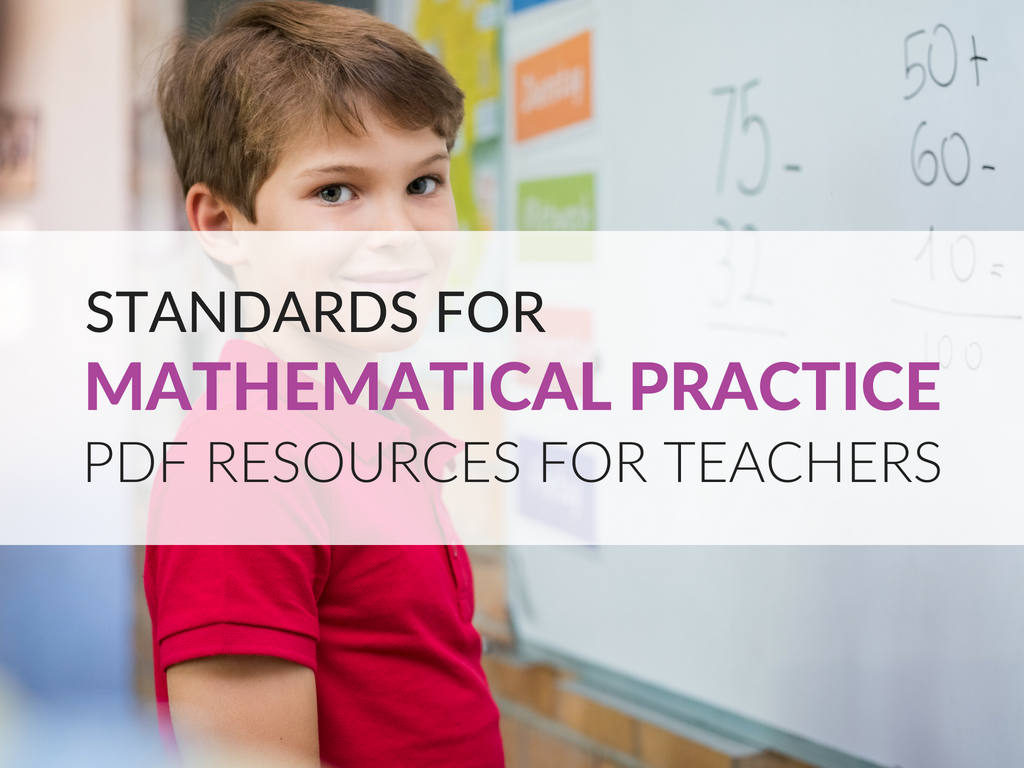The Standards for Mathematical Practice, sometimes called the math practice Standards, are part of the Common Core. Whether you are a fan of Common Core or not, these Standards will help your students think mathematically, conceptualize math, and become better problem solvers.

As math teachers head back to school, they can use these printables to remind students of the foundations for mathematical thinking and practice. Additionally, these resources are a great reference for teachers to go over to ensure their approach to teaching develops a more advanced mathematical understanding.

If your 2020-2021 school plans have you heading back into the classroom, many of the resources below can be hung in the classroom as anchor charts. The tip sheets and activities can be used in the classroom, or at home, by teachers and students to develop these practices.

If your 2020-2021 school plans have you teaching in the virtual classroom, have students print the anchor charts on 8.5 x 11 copy paper and then put them in the front of their daily math journals (use page protecters). Now they have fabulous reference sheets they can easily access during class or when completing homework! The tip sheets and activities can be used at home by students to develop these practices.

## Standards for Mathematical Practice Poster & Tip Sheet

I keep a simple list of the eight math Standards posted near my desk and in my plan book so I can refer to it often. I also print this list as a poster for my classroom walls so students and I can discuss them in class. Places that might be able to make the poster for you include your school department central office which may have a large format printer. If you cannot readily print a large poster, have students keep an 8.5 x 11 copy of the list in their daily math journals!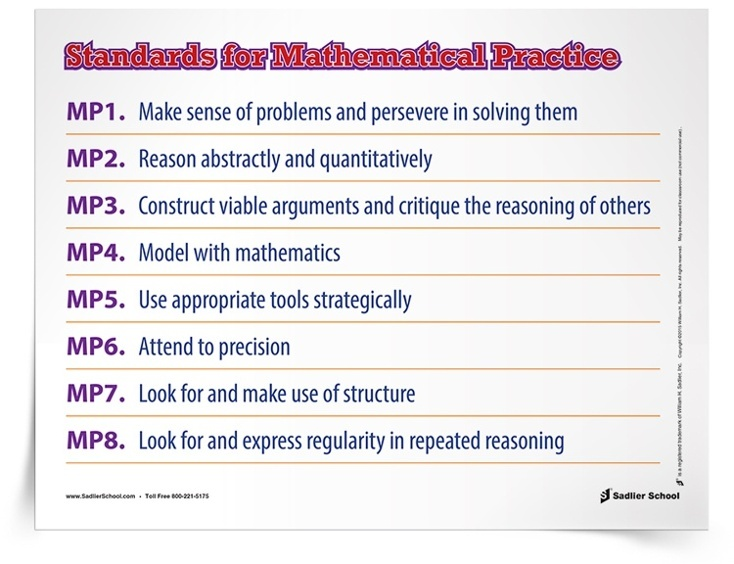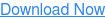## Make Sense of Problems & Persevere in Solving Them (MP1)

Mathematical Practice 1 serves as a way for students to structure their thinking and communication about a problem they need to solve. Although they do not include the mathematical content that we teach, they are useful Standards for the reasoning that students can use while we teach about content. These mathematical practices PDF posters use student-friendly language to break down what it means to make sense of problems and persevere in solving them for your students.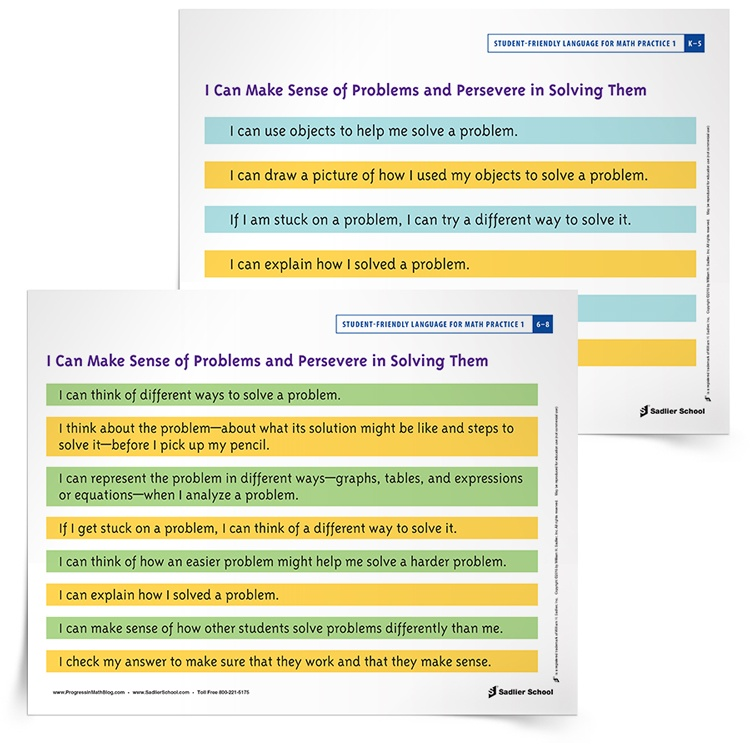## Reason Abstractly and Quantitatively in Middle School Tip Sheet (MP2)

The second of the Standards for Mathematical Practice is to “reason abstractly and quantitatively.”  At first glance, Mathematical Practice 2 can be one of the more confusing ones to understand. Essentially, when solving problems, it is important for students to first be able to make sense of the math in the problem, decontextualize, and then put the math back into the problem to contextualize it.

To make this standard easier to interpret, I break it down into three parts. I've turned these key elements of Mathematical Practice 2 into a tip sheet for the classroom. Download it to remind middle school students how to reason abstractly and quantitatively.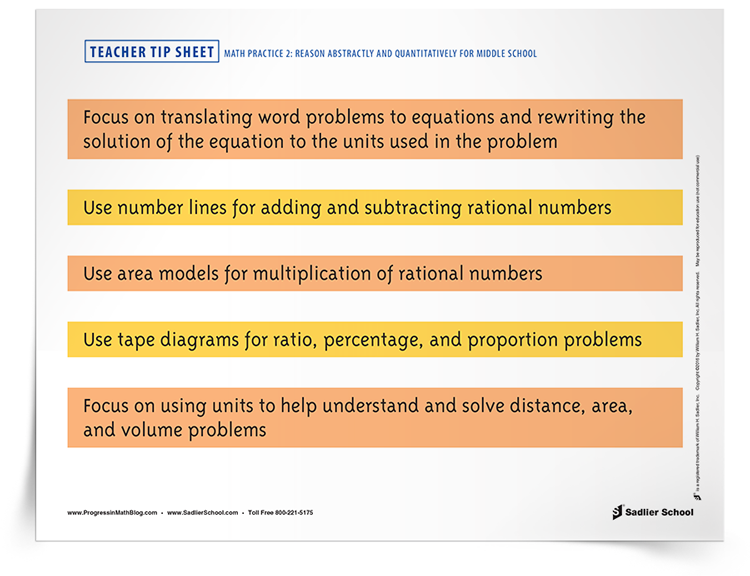## Abstract and Quantitative Reasoning Activities for K–3 (MP2)

The second mathematical practices PDF resource available for MP2 is for grades K–3. At a young age, students are expected to make sense of the meanings of quantities and their relationships and be flexible in the use of operations and their properties. With the Making Apple Ten Packs and Bunk Bed activities students will strengthen their abstract and quantitative reasoning.## Vocabulary to Construct Arguments and Critique Reasoning (MP3)

Focusing on vocabulary development will help students to construct viable arguments and critique the reasoning of others. To help you implement this in your classroom, download the poster of the conjunctive adverbs so students can refer to them as they learn to use Mathematical Practice 3.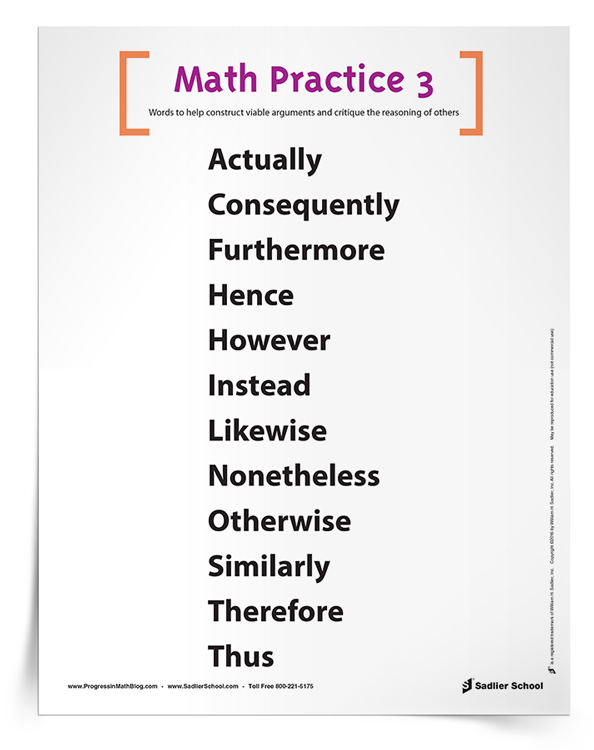## Rule of Four Template to Model with Mathematics in Grades 6–8 (MP4)

Mathematical Practice 4 requires students to apply mathematics in order to solve problems. One example of this standard is having students model a situation by writing an equation or inequality. Students need to understand the mathematical situation presented and translate it into an equation or inequality. Use the Rule of Four Templates to help students model with mathematics at the middle school level.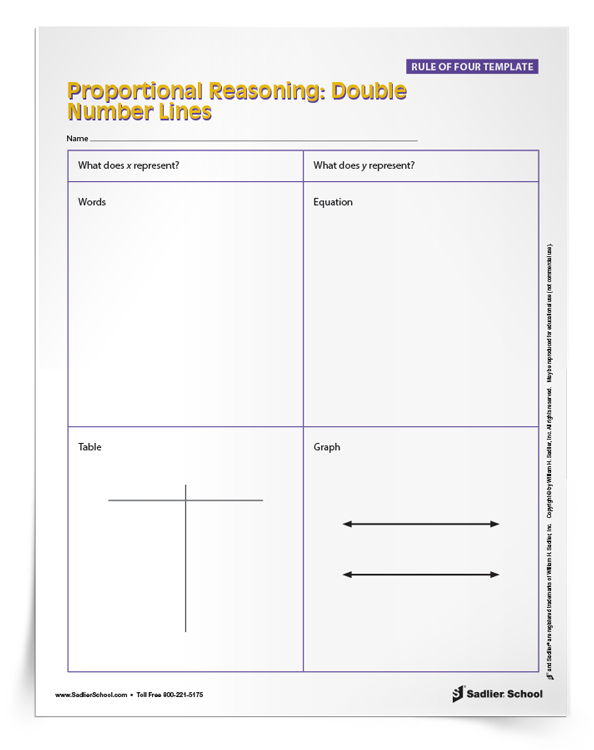## Using Appropriate Tools Strategically By Grade Level (MP5)

There is a great deal to say about how to use appropriate tools strategically, which is Mathematical Practice 5. It is simple to say that this Standard is about using a compass, protractor, ruler, or similar physical tool. There is so much more to using tools than just the use of physical objects! The Mathematical Practices PDF I have for download is a tip sheet with examples of tools you can put into practice. This tip sheet outlines choices you can use for teaching students to use appropriate tools strategically in each strand, at each grade level span. By giving students choices and talking with them about why they chose a specific tool, you can help them grow in their choice of strategies.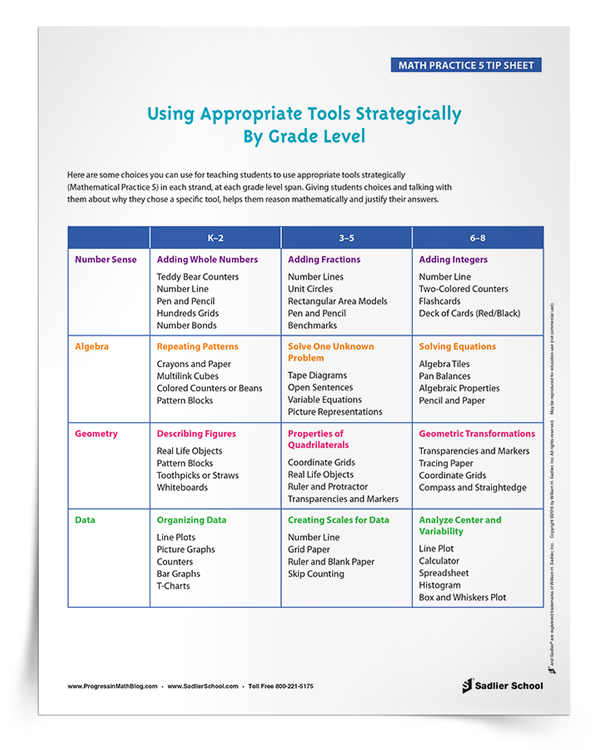## Attend to Precision Tip Sheet (MP6)

Mathematical Practice Standard 6 encourages students to focus on precision when solving and discussing math problems. This Standard should always be paired with MP1. Download a teacher tip sheet that highlights the key points of MP6, attend to precision, that can be implemented in the classroom.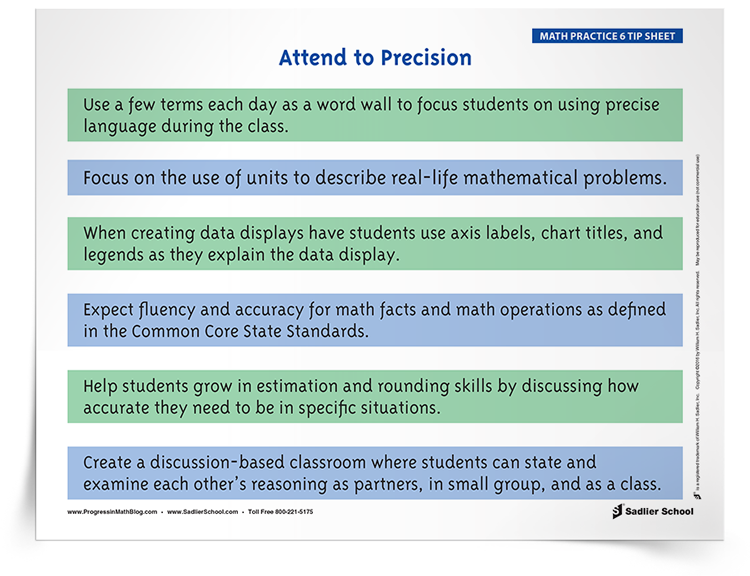## The Structure of Multi-digit Multiplication & Place Value Using an Area Model (MP7)

Mathematical Practice 7 helps students see the structure in mathematics– in our number system (place value) and the standard algorithms. Structure will show students that math makes sense. The next download will help your students see how the structure of the number system place value can be used to multiply two numbers. This method is a step in the process of the conceptual development of multiplication in Grade 4 that will help students eventually learn the standard algorithm for multiplying two two-digit numbers by the end of Grade 5. With this problem set (and answer key) students will see how place value works when computing multi-digit multiplication.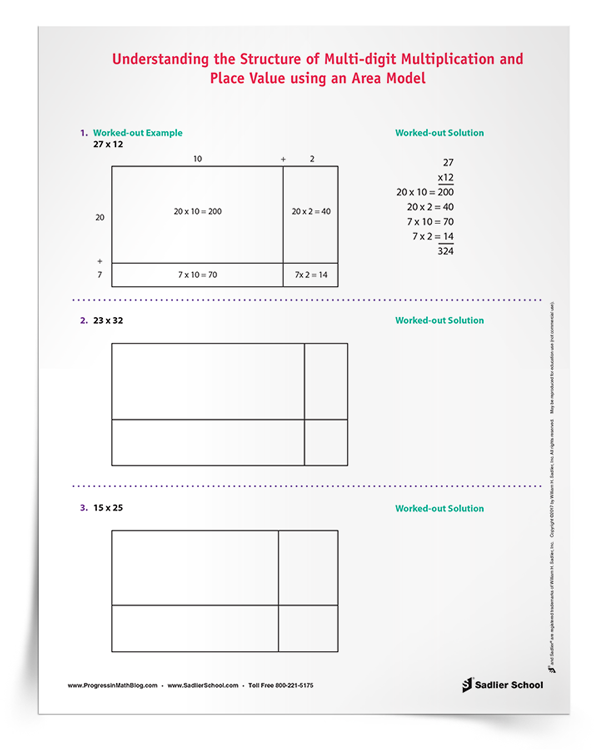## Problem-Solving Protocol to Explain Reasoning & Critique the Reasoning of Others (MP8, MP3)

In this era of increased demands on student reasoning in mathematics, I have been thinking about how to incorporate more student reasoning into my classroom. I came up with the P3: Partner Problem-Solving Protocol and Graphic Organizer! This protocol is great because it can be used at almost any grade level, with virtually any math topic. All you need is a set, or a couple of sets, of math problems to solve! Using the P3 Graphic Organizer students will work with a partner to solve math problems, explain their reasoning, and critique the reasoning of their partner.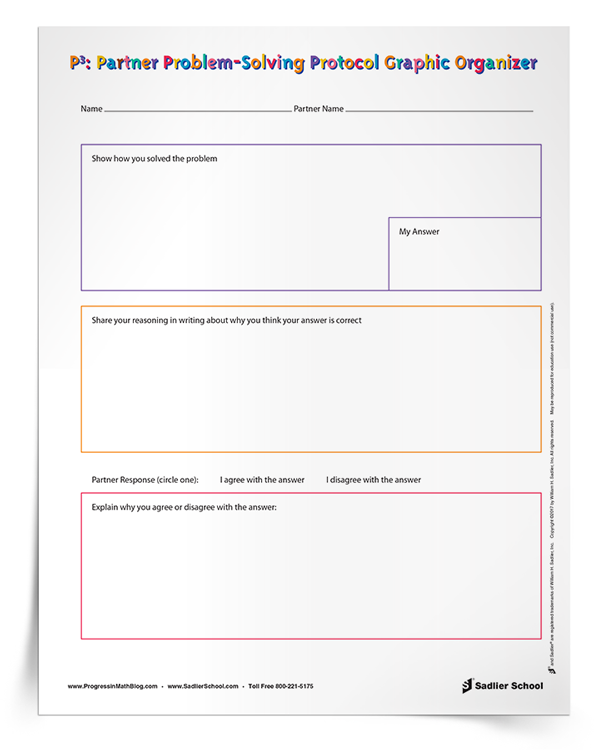## Tips for Implementing the Mathematical Standards

The Standards for Mathematical Practice should be an integral part of your daily math lessons. These Standards describe what students should be thinking and doing while learning mathematics, thereby helping them conceptualize mathematics. Here is a tip sheet with suggestions for implementing the eight Standards for Mathematical Practice into your math classroom.